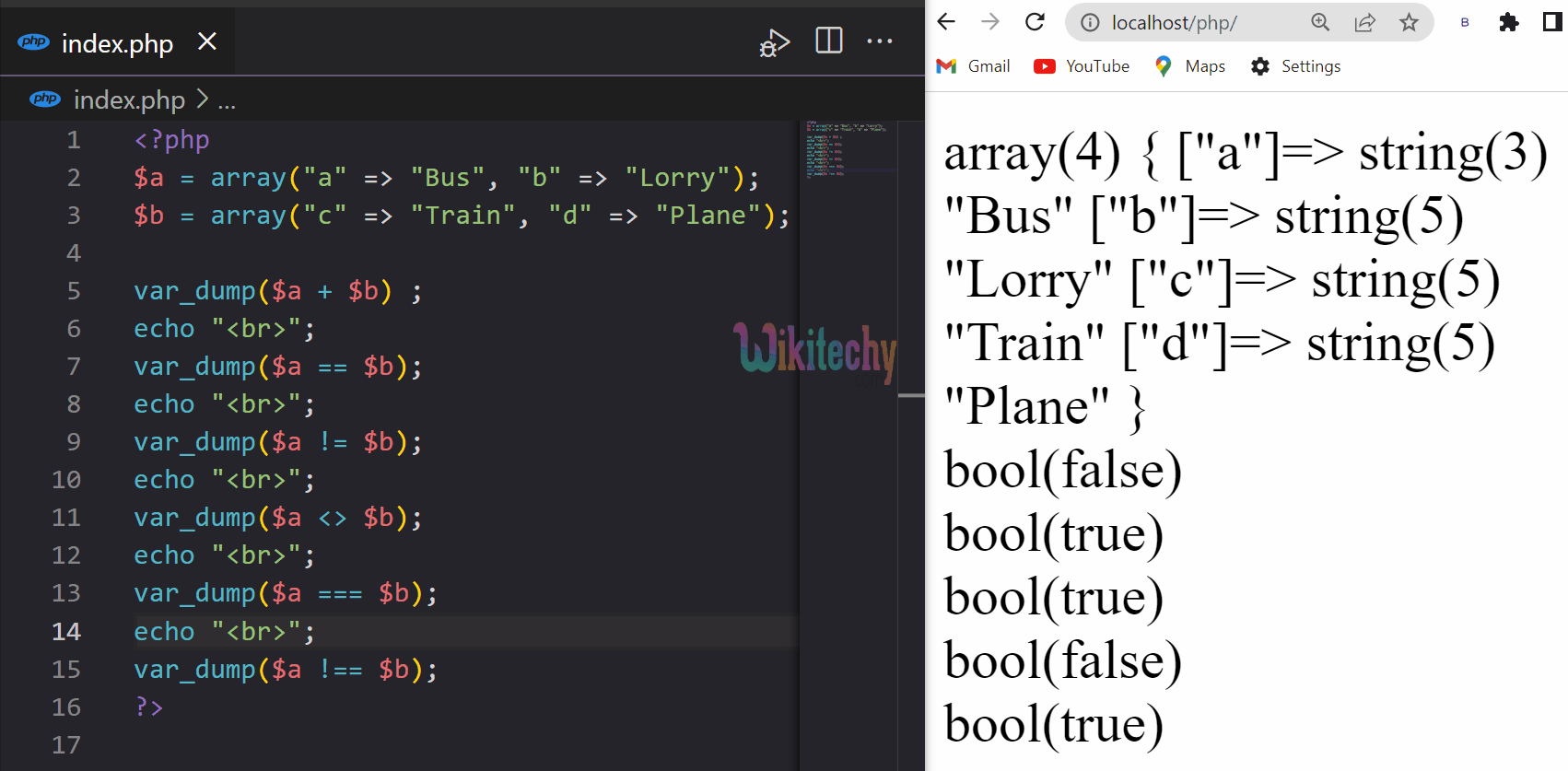# PHP Array Operators - Array Operators in PHP

## PHP Array Operators

• PHP array operators are used to compare arrays and to determine the relationship between them.
• They are used to compare values in arrays, check if a certain key or value exists in an array, and to merge two arrays together into one.
Operator Name Syntax Operation
+ Union \$a + \$b Union of both i.e., \$a and \$b
== Equality \$a == \$b Returns true if both has same key-value pair
!= Inequality \$a != \$b Returns True if both are unequal
=== Identity \$a === \$b Returns True if both have the same key-value pair in the same order and of the same type
!== Non-Identity \$a !== \$b Returns True if both are not identical to each other
<> Inequality \$a <> \$b Returns True if both are unequal